Decomposing Functions -- Tutorial

In each of the problems below, for a given function h(x) find its decomposition into simpler functions:

h(x)=f(g(x)) .

Example. For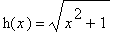, we can take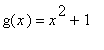and.

We can also go with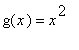and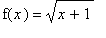.

Usually, there is more than one correct answer.

Problem 1. Find two possible decompositions of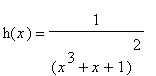.

Click below to see the answer. Make the answer window smaller by clicking on the middle icon of its upper right corner. To close the answer window and get back to the quiz, click on the X in the upper right corner of the answer window. Close one answer window before opening another.

>

Problem 2. Find one decomposition of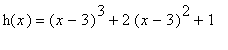.

>

Problem 3. Find two possible decompositions of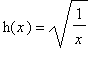.

>

Problem 4. Find one decomposition of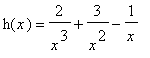.

>

Problem 5. Find one decomposition of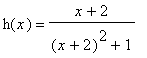.